# Divide money 2

Ben and Dan had the same amount of money at the start. When Ben gave 300 to Dan, the ratio of Ben 's money to Dan's money became 2:3. How much money did each have at first?

x =  1500

### Step-by-step explanation:

3(x-300) = 2(x+300)

3•(x-300) = 2•(x+300)

x = 1500

Our simple equation calculator calculates it.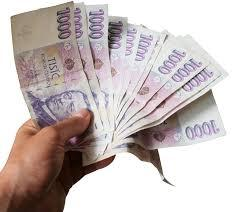We will be pleased if You send us any improvements to this math problem. Thank you!Tips to related online calculators
Check out our ratio calculator.
Do you have a linear equation or system of equations and looking for its solution? Or do you have a quadratic equation?

## Related math problems and questions:

• Bike cost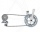The father gave his son € 100 to buy a bicycle, which was 40% of the total amount of the bicycle. How much did the bike cost?
• Divide moneyDivide 1200 USD at a ratio of 1:2:3:4:5:6:9:10
• Clothes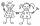Danka and Janka collect clothes. Danka had 9 more than Janka, so she gave her 7. Which now has more cloth and how many?
• Three workersThree workers got paid for work. First earn € 50, the second € 100 and third € 60. For further work should receive together € 735. How do you divide that amount in order to be distributed in the same proportion as the first job?
• String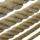Divide the string length 800 cm in ratio 1: 5
• Jan and DanJan and Dan had the same money. Jan bought 5 workbooks and left him 15 CZK. Dan 6 and left him nothing. How much money have in total?
• Honza + Alice + Tonda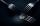Honza + Alice + Tonda have a total of 111 USD. The ratio between Honza and Alena is 5:6 and the ratio between Alice and Tonda is 4:5 How many money have each of them?
• TwelveTwelve students work on a week forestry brigade. One hundred spruces will receive x CZK, one hundred pine y CZK. How many receive each one students did in one day if they planted a total of 25,000 spruces per week and 30,000 pine trees? Express by express
• Summerjob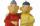Albert, Beny, Cyril and Dan worked on summerjob. From the total revenue Albert received 2/5, Beny 1/6 , 3/10 Cyril and Dan the rest which is 324 euros. How many euros earned every one of them?
• Divide 5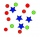Divide 288 in the following ratio 3 : 4 : 5
• Money split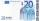Anton and Ferko got 2,500 euros together. The amount was split in a 1:4 ratio. How much did each get?
• Water tank300hl of water was filled into the tank 12 m long and 6 m wide. How high does it reach?
• The inheritanceDad originally decided to bequeath the money to his two sons so that they would share it 8: 7 (older and younger). Then he changed his mind and divided the same amount in the ratio 11: 9 (older and younger). This increased the value of the inheritance for
• Natural gas in kWh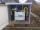Gas consumption for 2017 was 11,301 kWh I paid 532 € How much 1 m3?
• Money 6Lita had some money. She spent 1/2 of it on a hand bag and 1/3 of the remainder of the blouse. She had 260.00. How much money did she have at start?
• Dividing moneyMilan divided 360 euros in the ratio 4: 5, Erik in the ratio 500: 625. How many euros did the individual parts of Milan and how many Erik?
• Moneys in triple ratioMilan, John and Lili have a total 344 euros. Their amounts are in the ratio 1:2:5. Determine how much each of them has?